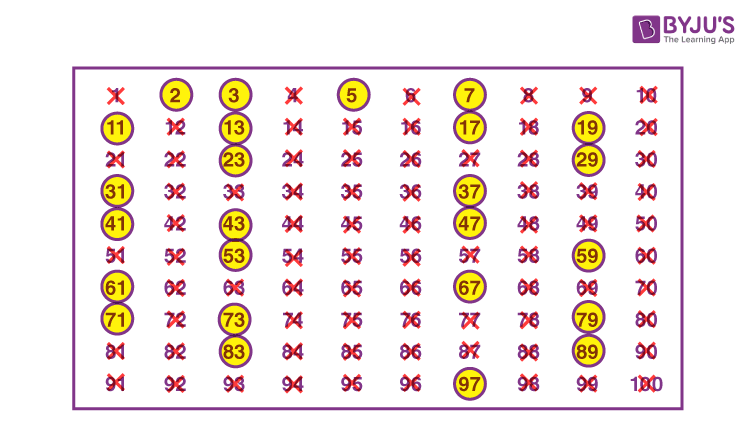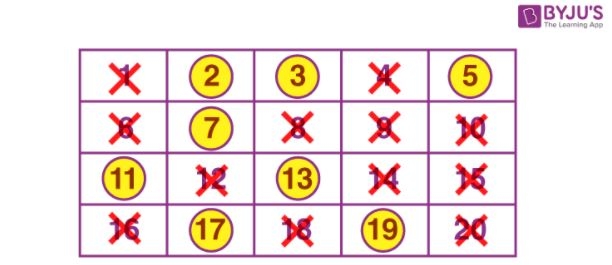# Sieve of Eratosthenes

Sieve of Eratosthenes is a method to find the prime numbers and composite numbers among the group of numbers. This method was introduced by Greek Mathematician Eratosthenes, in the third century B.C.

Also read: How to Find Prime Numbers?

In Mathematics, there are various methods to find the prime and composite numbers such as factorisation and division methods. But in the case of the Sieve of Eratosthenes method, it is easy to list down the prime numbers among a set of numbers in a quick way. Class 6 students can learn this method here and excel in it.

## What are Prime and Composite Numbers?

Before we proceed to learn about the Sieve of Eratosthenes method, let us first learn the definitions of prime and composite numbers.

### Prime numbers

The numbers apart from 1 whose only factors are 1 and the number itself are known as prime numbers.

For example: 2 has only two factors: 1 and 2. Apart from that, 2 is not evenly divisible by any other factor. In the same way, 3, 5, 11, 17, etc., are primes.

### Composite numbers

The numbers that have more than two factors are called composite numbers. Therefore, the composite numbers are evenly divisible by two or more factors.

For example, 6 is a composite number because it has a total of four factors, i.e. 1, 2, 3, and 6. Hence, 6 is exactly divisible by all its factors.

 Facts: 2 is the smallest prime number which is even Every prime number except 2 is odd

## Sieve of Eratosthenes – Prime Numbers 1 to 100

Now here we are going to encircle the prime numbers from 1 to 100 using the Sieve of Eratosthenes method. As we have already discussed the prime and composite numbers above, therefore, it will be more convenient to find the difference between them.

The steps involved to separate the prime numbers from 1 to 100 are as follows:

Step 1: First write all the natural numbers from 1 to 100, row-wise and column-wise as shown in the below figure.

Step 2: Put a cross over 1, as it is neither a prime number nor a composite.

Step 3: Now, encircle the number 2 (which is a prime number) and cross all the multiples of 2, such as 4,6,8,10,12, and so on. Since, all the multiples of 2 are composite.

Step 4: Next, encircle the number 3, and put a cross over all the multiples of 3, such as 6,9,15,21,etc. Since apart from 3 all its multiples are composite.

Step 5: Again, encircle the number 5 (since it has only two factors), and put a cross over all the multiples of 5.

Step 6: Now encircle 7 and cross all the multiples of 7

Step 7: Encircle 11 and cross all the multiples of 11

Step 8: Continue the process unless all the numbers are either encircled or crossed.Therefore, we have concluded that all the encircled numbers are prime numbers and all the crossed numbers are composite numbers. So, the prime numbers from 1 to 100 are:

 2, 3, 5, 7, 11, 13, 17, 19, 23, 29, 31, 37, 41, 43, 47, 53, 59, 61, 67, 71, 73, 79, 83, 89, and 97

Hence, the Sieve of Eratosthenes method is completed.

## Solved Examples

Q.1: Find if 101 is a prime number or not.

Solution: 101 is divisible by only two numbers, 1 and 101. Therefore, 101 is a prime number.

Q.2: What are all prime numbers less than 20. (Use the Sieve of Eratosthenes method).

Solution: Let us first write the numbers from 1 to 20.

 1 2 3 4 5 6 7 8 9 10 11 12 13 14 15 16 17 18 19 20
• Now, exclude 1, since it is not a prime nor a composite number.
• In the next step, encircle 2 and cross the multiples of 2 (4, 6, 8, 10, 12, 14, 16, 18, 20)
• Encircle 3 and cross the left multiples of 3 (9, 15)
• Encircle 5 and cross the left multiples of 5 (only 10 is left)
• Now, encircle the rest numbers 7, 11, 13, 17 and 19.Therefore, the required prime numbers between 1 to 20 are 2, 3, 5, 7, 11, 13, 17 and 19.

Q.3. Express the number 21 as the sum of three prime numbers.

Solution: 21 can be written as the sum of three prime numbers:

21 = 3 + 5 + 13

Where 3, 5 and 13 are prime numbers.

### Practice Questions

1. Write all the prime numbers between 1 to 50 using Sieve of Eratosthenes method.
2. Express 61 as the sum of three primes.
3. Write all the composite numbers less than 30.
4. Give three pairs of prime numbers whose difference is 2.

## Frequently Asked Questions on Sieve of Eratosthenes

### What is the Sieve of Eratosthenes method for?

Sieve of Eratosthenes method is to find the prime numbers among groups of numbers.

### How to find prime numbers using the Sieve of Eratosthenes method?

Sieve of Eratosthenes method is easy to use. We need to cancel all the multiples of each prime number beginning with 2 (including the number 1, which is not a prime or composite) and encircle the rest of the numbers. The encircled numbers will be the required prime numbers.

### Is 11 a prime number?

Yes, 11 is a prime number, as it has only two factors 1 and the number itself.

### What is the greatest prime number between 1 to 10?

The greatest prime number between 1 to 10 is 7.

### 2 is the only even prime number. True or False.

True, 2 is the only even prime number, rest all the prime numbers are odd numbers.